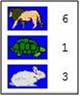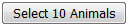# 8.15: Sampling Demonstration

Learning Objectives

• Distinguish between simple random sampling and stratified sampling.
• Describe how often random and stratified sampling give exactly the same result.

## Instructions

The sampling simulation uses a population of $$100$$ animals: $$60$$ lions, $$30$$ turtles, $$10$$ rabbits.

### Options: This option allows you to draw a sample of $$10$$ animals at a time with each animal having an equal chance of being selected.: This option allows you to draw a sample of $$10$$ animals at a time, with each number of animals from a group being proportional to their group’s size of the population.

### Simulation ResultsFigure $$\PageIndex{1}$$: Simulation Results

The number of animals chosen from each group when a sample is drawn is shown next to the picture of the animal.

## When you give it a try

### Random Sampling

• Begin by leaving theoption selected.
• Click on thebutton, $$10$$ animals will be selected out of the population.

Note: The animals become highlighted in blue and a number count of each animal selected will be listed by each animal image.

• Each time you push thebutton, another sample will be drawn and the new tally will be shown on the right side of the previous sample.
• You should get different tally results for each animal as you select, however the computer may give you the same number drawn from an animal category every now and then.

### Stratified Sample

Note: Your animals should become highlighted in blue and a number count should be listed by each animal image.

• Click on thebutton, to clear the simulation.
• Select theoption.
• Click on thebutton a few times.
• As you get a new tally for everybutton, notice that the number of animals stays the same, but the animals selected are not always the same animals.

## Illustrated Instructions

The opening screen of the sampling simulation displays all $$100$$ animals in the population. You can select between a random sample and a stratified sample directly below the population and then generate a sample of ten animals.Figure $$\PageIndex{2}$$: Sample Choices

Below is an example of a random sample. Notice that animals selected are highlighted in the population and the total number of animals selected from each category is listed at the bottom of the simulation.Figure $$\PageIndex{3}$$: Random Sample